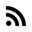# Always cast. A Left-shift story

I was working on a small project with a Texas Instruments microcontroller.

The MSP430FR2433.

I wanted to convert an array with hex values to its proper integer value.

For example, if I had a two-byte array like this:

``````| 0x01 | 0x00 |
``````

That would be 256 (“0x0100”).

All the integer values were `unsigned`. In particular `uint32_t`. However, I found a bug when the array is `| 0x89 | 0x35 |`. I got 4294936885 instead of 35125.

So.. what’s the difference between `| 0x89 | 0x35 |` and `| 0x01 | 0x00 |` ? BTW, `| 0x01 | 0x00 |` works.

To find it out … keep reading.

# Setup of the function

• Inputs:
• An array with “hex” values. `uint8_t *hex_array`.
• Array length. `uint8_t len`.
• Output:
• An unsigned integer of 32 bits. `uint32_t`

The function went as follows:

``````uint32_t usart_hex_array_to_u32(uint8_t *hex_array, uint8_t len)
{
uint32_t sum = 0;
uint8_t displacement = 0;;

for( uint8_t i=0; i<len; i++)
{
uart_debug("i:%i %i",i, hex_array[i]);
displacement = 8*(len - 1 - i);
sum += ( hex_array[i] << displacement );
}

return sum;
}
``````

# Assumptions

On this line `sum += ( hex_array[i] << displacement );` Before actually the Left-shift operator («) take part. The C compiler does an implicit casting.

So. The compiler casts `hex_array[i]` from `uint8_t` to `uint32_t`. Because `sum` is `uint32_t`.

And also the compiler casts `displacement` to `uint32_t`.

This is because the compiler does an Implicit Conversion or Data Promotion when in an expression more than one data type is present.

## Implicit Conversion or Data Promotion

The goal of Implicit Conversion is to avoid loss of data.

The gcc compiler has the following hierarchy or ranking.

``````/* From         ->  To*/
bool            -> char
char            -> short int
short int       -> int
int             -> unsigned int
unsigned int    -> long
long            -> unsigned
unsigned        -> long long
long long       -> float
float           -> double
double          -> long double
``````

In this post Details of C that every noob forgets . You can read more about promotions.

# Debugging

At the beginning I said I found a bug when the array was `| 0x89 | 0x35 |`. I got 4294936885 instead of 35125.

The same applies when the array is `| 0xD1 | 0xC3 |`. I got 4294955459 instead of 53699.

The error laid at the implicit data conversion.

Because when I casted `hex_array[i]` to `uint32_t`. It worked.

``````uint32_t usart_hex_array_to_u32(uint8_t *hex_array, uint8_t len)
{
uint32_t sum = 0;
uint8_t displacement = 0;;

for( uint8_t i=0; i<len; i++)
{
uart_debug("i:%i %i",i, hex_array[i]);
displacement = 8*(len - 1 - i);
sum += ( ( (uint32_t)hex_array[i]) << displacement );
}

return sum;
}
``````

# The Experiment

When the array input was `| 0x89 | 0x35 |`. The output was 4294936885.

4294936885 to hex was `0xFFFF8935`.

Aha!….

In theory, I got the “right value”. Because `0x8935` was 35125. The expected value.

But the question is… Why `0xFFFF8935`?

Let’s check the Data Promotions. `char -> short int -> int -> unsigned int`

In the embedded world it would be `uint8_t -> int16_t -> uint16_t -> int32_t -> uint32_t`

Look!

Data promotion always goes from unsigned type with fewer numbers of bits to signed type with more bits. Example `uint8_t -> int16_t`.

So…

The compiler casts `0x89`(`0b10001001`) to `int16_t`.

NOTE: Compiler uses 2’complement to represent signed integers. In 2’complement `0x89` is -119. For the compiler -119 should stay as -119 after promotion. So -119 as `int16_t` is `0xFF89`.

NOTE: Compiler DOESN’T use 2’complier to represent unsigned integers. So, `0xFF89` as `uint16_t` is still `0xFF89`.

At the end -119 as `int32_t` is `0xFFFFFF89`.

When the code executed `hex_array << 8`.. it performed `0xFFFFFF89 << 8`. That’s why the result was then `0xFFFF8900`.

At the end I got 4294936885 (`0xFFFF8935`).

# Why casting works

When I did `(uint32_t) hex_array[i]`. What I was saying was that `hex_array[i]` was direct a 32-bit value.

For example, if `hex_array[i]` were `0x89`. Then `(uint32_t)hex_array[i]` would be `0x00000089`.

# Conclusion

Practice defense C programming. With this bug, you learned to avoid implicit casting.

Always cast!